General Question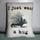When something is considered infinite what does that encompass?

Asked by tacres (559) May 4th, 2012

I am not really sure how to formulate this question but here it goes.
When calling something infinite does that include mass, time, size,
distance. Can these be defined as infinites separently? Remember when we were pretty sure the atom was as small as you could go? Then it was discovered there were quarks etc. Can smallness be infinite ( do you just end up with nothing) or is size only able to be infinitely larger? My mind locks up when I try to get my head around infinity. So Collective what is infinity?

Observing members: 0Composing members: 0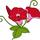Infinity is infinite. The human mind cannot compute depth and spaciousness without end.
Infinity is space within space within space.
Every atom is a black hole, so yes, smallness can be infinite.

Coloma (47125)“Great Answer” (1) Flag as…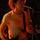Infinite is not so much a measurement, as it is an arbitrary name given to the sum of all things (or one thing). If we discover some new measurement we didn’t include the last time we called something infinite, then infinite automatically extends to include it.. because it is just an arbitrary name for everything.

tedd (14073)“Great Answer” (1) Flag as…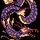Infinity is a mathematical concept. It’s not clear at all that infinite quantities exist in the real world.

In fact quantum mechanics would seem to disprove the notion of infinitesmals, and much of the work in modern cosmology points to a finite universe.

Mathematically speaking, infinity is described in set theory. An infinite set is, by definition, a set where the whole is not necessarily greater than its parts. The fun part is that there are actually different “sizes” of infinity. For example, the set of integers is “countably infinite.” But the set of real numbers is “uncountably infinite.” Real numbers are infinite to a greater degree than integers.

Qingu (21175)“Great Answer” (6) Flag as…More on infinite sets, because this is actually a really weird concept.

Take the set of natural numbers (1, 2, 3, and so on). This set is infinite.

This set includes a supposedly smaller set of numbers—the even numbers (2, 4, 6, and so on).

But here’s the thing: there as many even numbers as there are natural numbers. Every natural number can be “matched up” with an even number. Both sets are infinite to the same degree.

This seems like a paradox, right? How can there be just as many even numbers as natural numbers when clearly there should be half as many? Well it turns out that it’s a feature of infinite sets, not a bug. Georg Cantor was the first to discover this. If you define an infinite set as one where its subset (even numbers, for example) is just as large as the entire set, then suddenly you have a comprehensible way to talk about infinite sets. (“Comprehensible” in terms of higher mathematics, of course. It sure doesn’t make sense to me. But it works, so we use it.)

Qingu (21175)“Great Answer” (4) Flag as…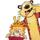I don’t understand infinity. But if it didn’t exist I would be even more baffled.. wondering what was on the outside of “the end of things”.

digitalimpression (9915)“Great Answer” (1) Flag as…I understand about numbers being infinite& the whole mathmatical concept. Well not really but in very basic generalities I do. What I ponder when driving in circles on my tractor in my hay field is if the universe is finite what could possibly be outside of that? I realize that I will never get an answer in my life time or in perhaps an infinite number of lifetimes so it is very much an open ended question. But I still wonder. I can’t help but also wonder if our universe & all the goodies within are not part of a larger body that we are just not aware of.
We could just be a cell on some huge beings body. Maybe we’re bacteria!
If I understand correctly the universe is expanding but there is evidence that the expansion is not slowing down but speeding up. So what is it expanding into? Is the area that it is growing into already there? Or is it being created as it grows?
I have to stop my head is spinning.

tacres (559)“Great Answer” (0) Flag as…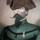The exact definition of “infinite” seems to vary depending on what you’re measuring. An infinite amount of time, or space, wouldn’t really have a correspondingly infinite mass, for example.

AFAIK the only thing that we can conclude about what the expanding universe is expanding into is that it’s not universe, though popular theory suggests that there are other universes in the neighborhood.

Nullo (21978)“Great Answer” (1) Flag as…As I understand it, the evidence points to an infinite Universe, but a finite Universe is not that difficult to conceive of. Imagine a two dimensional universe on the surface of a sphere. The inhabitants would see no boundaries and if they travel far enough along what they think of as a straight line they will return to their starting point.

Similarly, our universe could be equivalent to the three dimensional surface of a four dimensional object.

LostInParadise (29287)“Great Answer” (1) Flag as…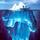People sometimes use “infinite” as a euphemism for a very large quantity, such as the number of water molecules in the ocean, or poetically such as “infinite love.” As others have pointed out, infinity is a very different concept from merely numerous but finite.

Something that is “infinitely small” is better described as “infinitesimal,” such as the quantities in calculus that converge to a limit of zero. “Finite” is used to distinguish something which is neither infinite nor infinitesimal.

These are mathematical concepts not connected to physical reality. In physics practically everything is finite at both ends of the scale. We’re not sure whether the universe is finite or infinite, but there is reasonable evidence for being finite. At decreasing scales, we observe electrons and quarks to be point-like particles with no physical extent, but quantum theory puts limits on measurable smallness. In some theoretical models (not yet validated) all of physical reality is subject to quantization at the very smallest scales – akin the pixel limit of images.

gasman (11315)“Great Answer” (1) Flag as…@LostInParadise What you describe is usually referred to as “finite but unbounded” – yet not infinite. In such a universe if you look far enough into the distance you can see the back of your head!

gasman (11315)“Great Answer” (1) Flag as…Response moderated (Spam)
Response moderated@gasman Would your theoretical gaze necessarily continue along the same vector, or could you get a profile view of yourself?

Nullo (21978)“Great Answer” (1) Flag as…or